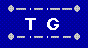Interesting Math Problems Home  |  Send Your Feedback

This is a collection of interesting math problems, taken mostly from Yahoo!Answers.

 COMBINATIONS: ALGEBRA: ANALYTIC GEOMETRY and CALCULUS: NUMBER THEORY: CRYPTARITHMS: RECURRENCE RELATIONS: INDUCTION: PROBABILITY: GEOMETRY: LOGIC: GRAPH THEORY: OTHER: